# RD Sharma Solutions for Class 11 Chapter 17 - Combinations Exercise 17.2

Here in this section, we intend to discuss some problems in real life where the formula for combinations, C(n,r) and its meaning can be applied. The solutions are formulated by expert tutors in an interactive manner to create interest in learning among students. These problems help students in improving their conceptual knowledge, which is significant from the exam point of view. In order to get a good command over the topics discussed under this chapter, students can make use of RD Sharma Class 11 Maths Solutions pdf, from the links given below.

## Download the pdf of RD Sharma Solutions for Class 11 Maths Exercise 17.2 Chapter 17 – Combinations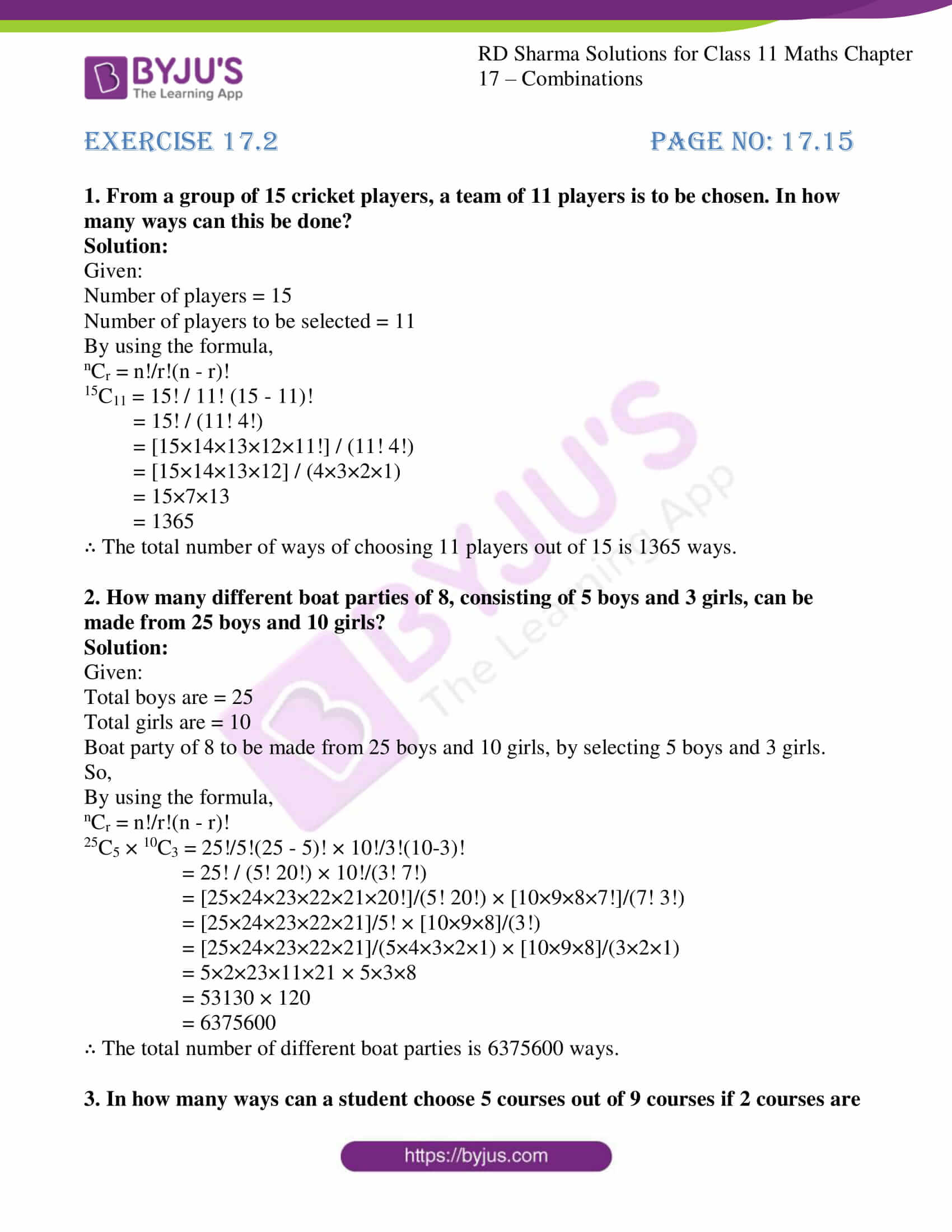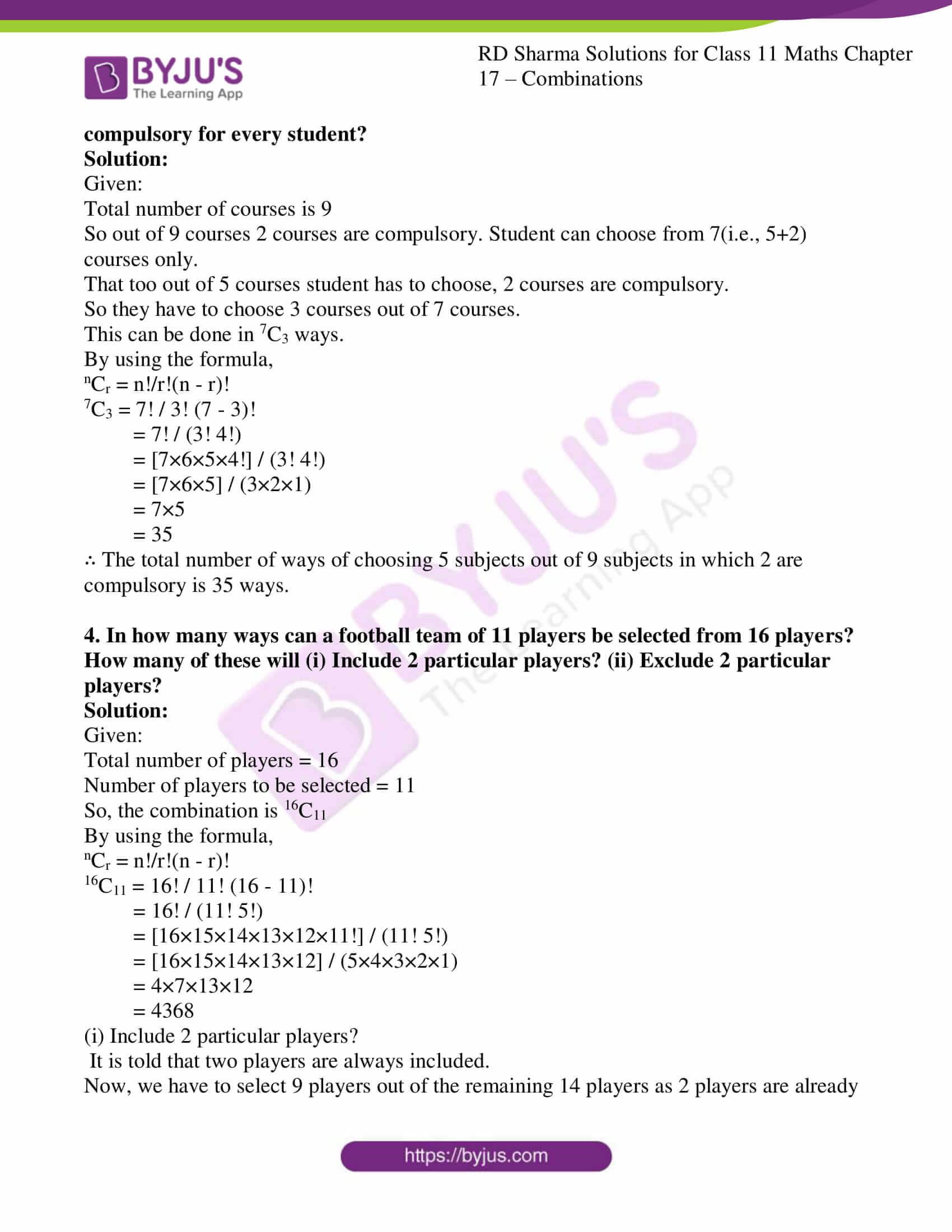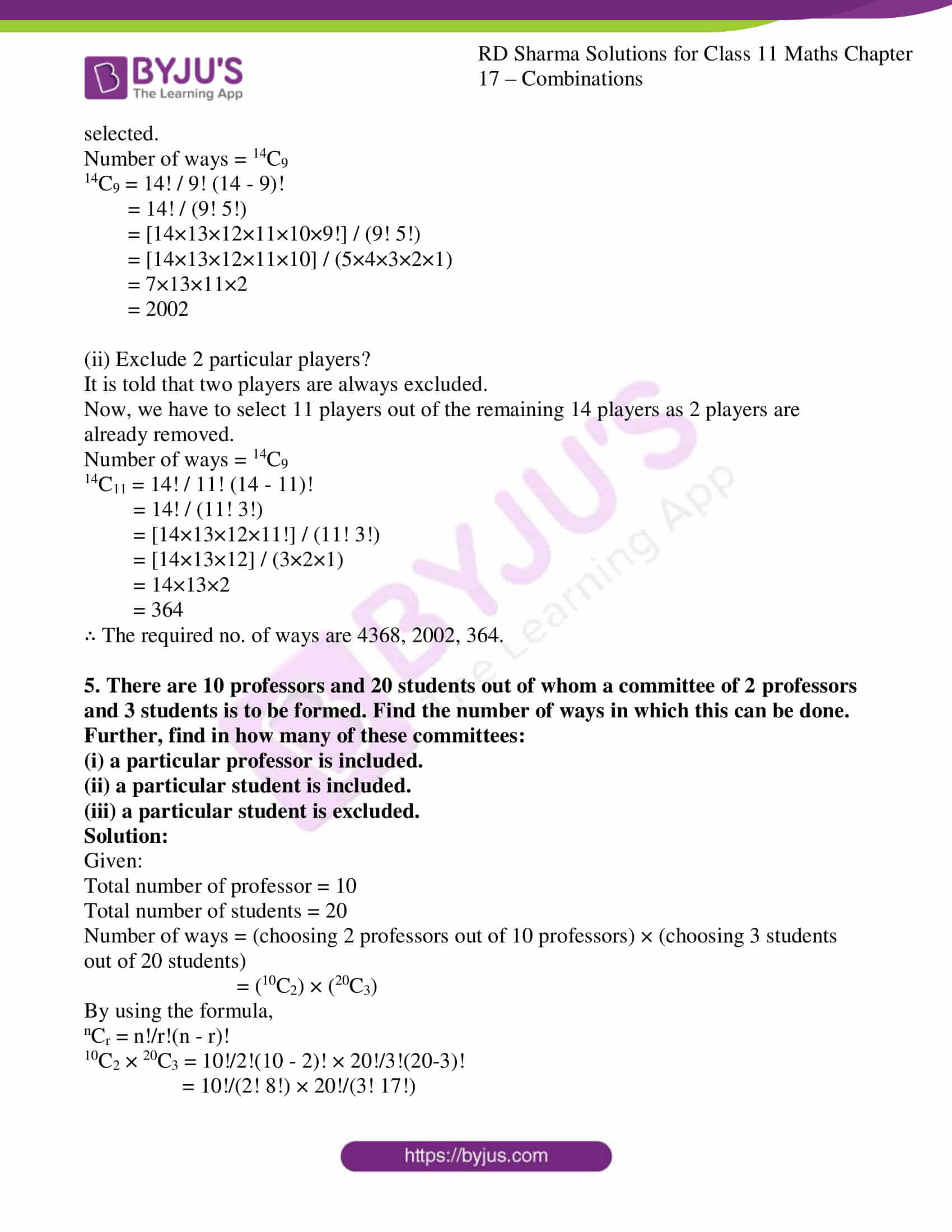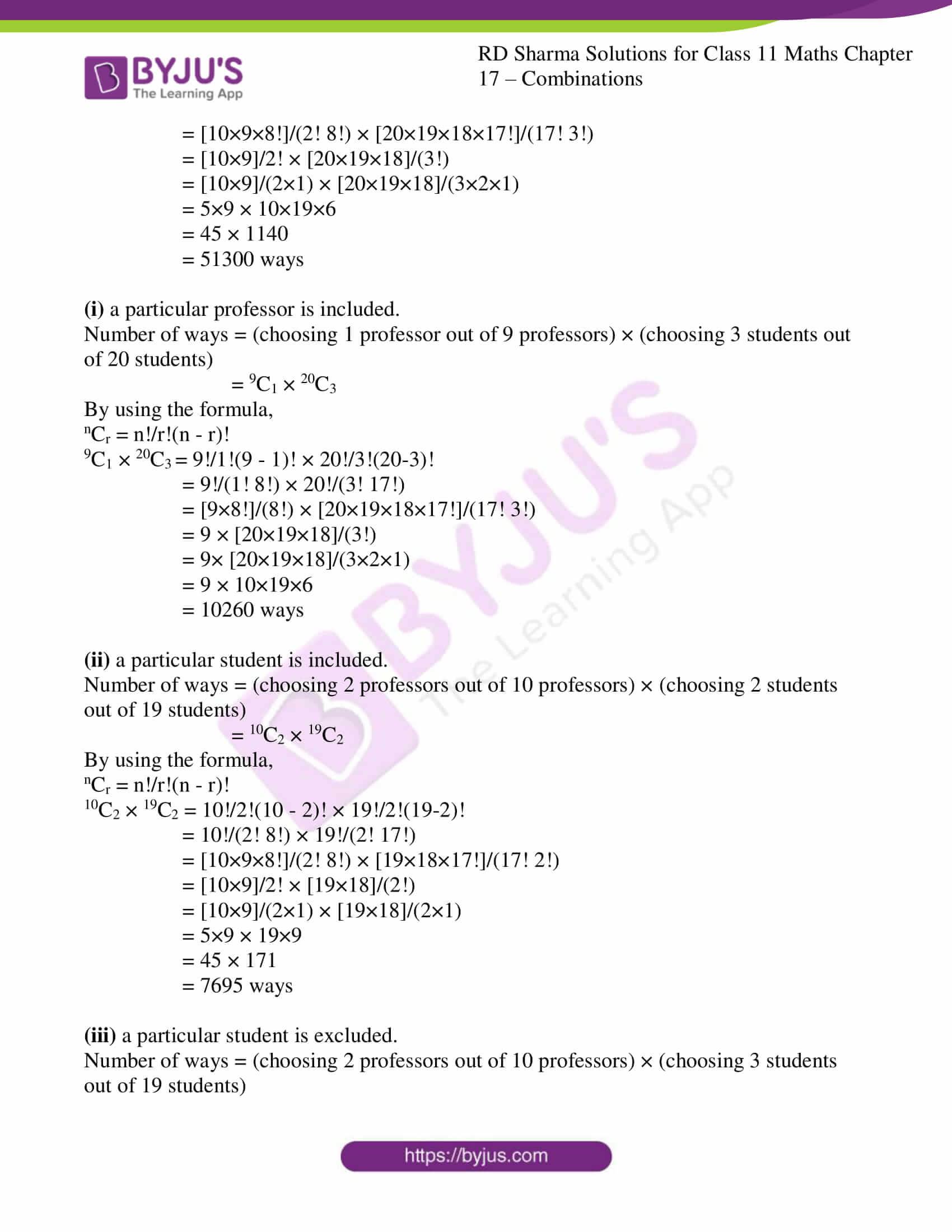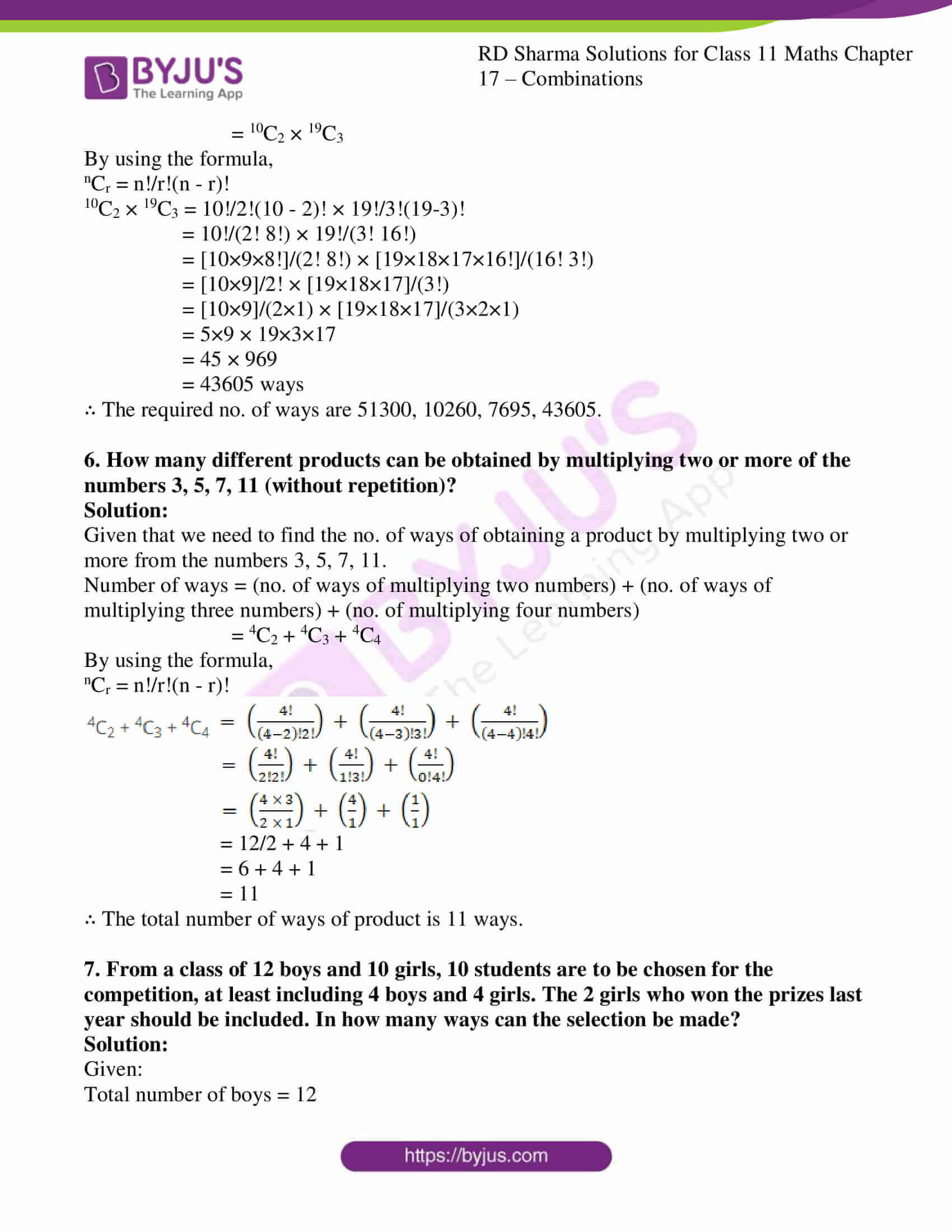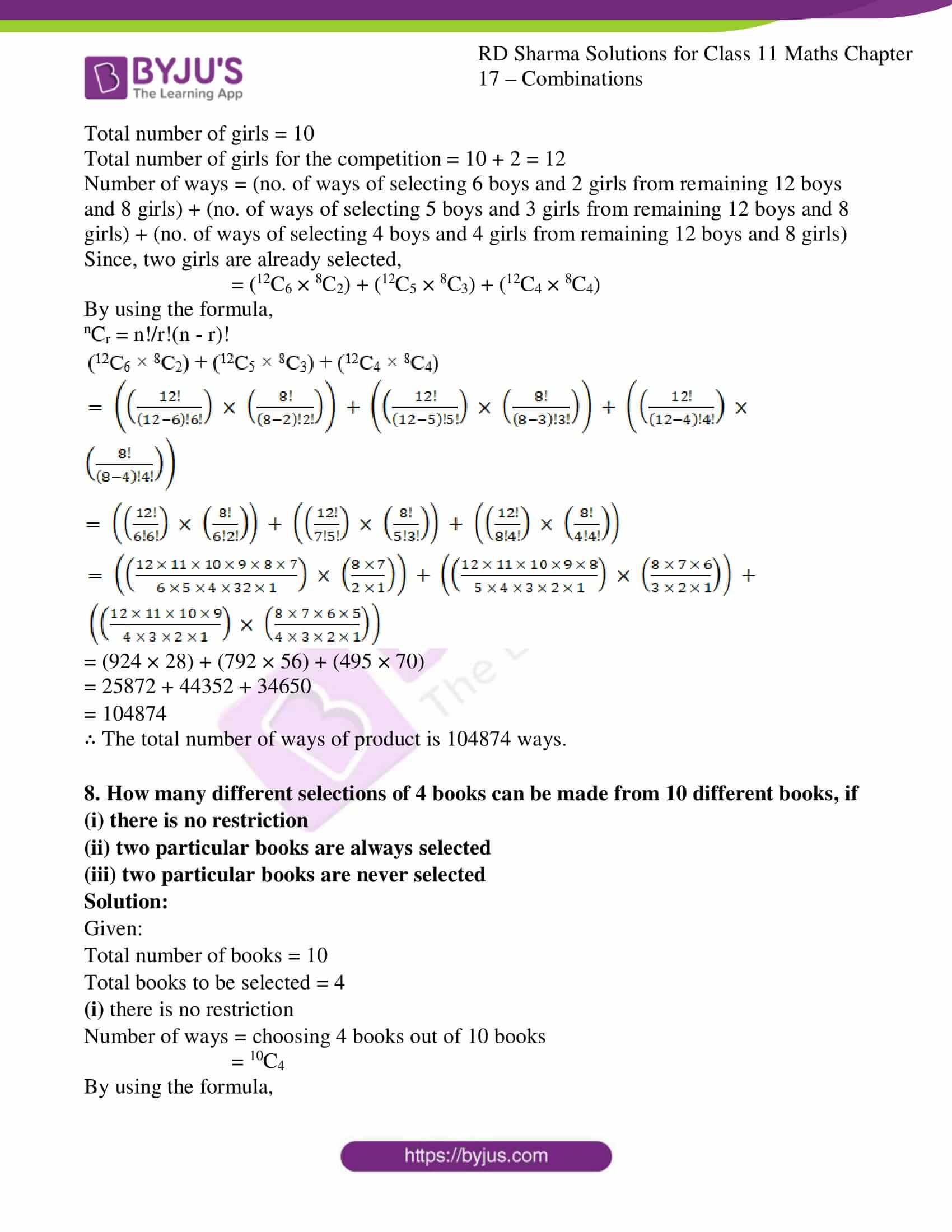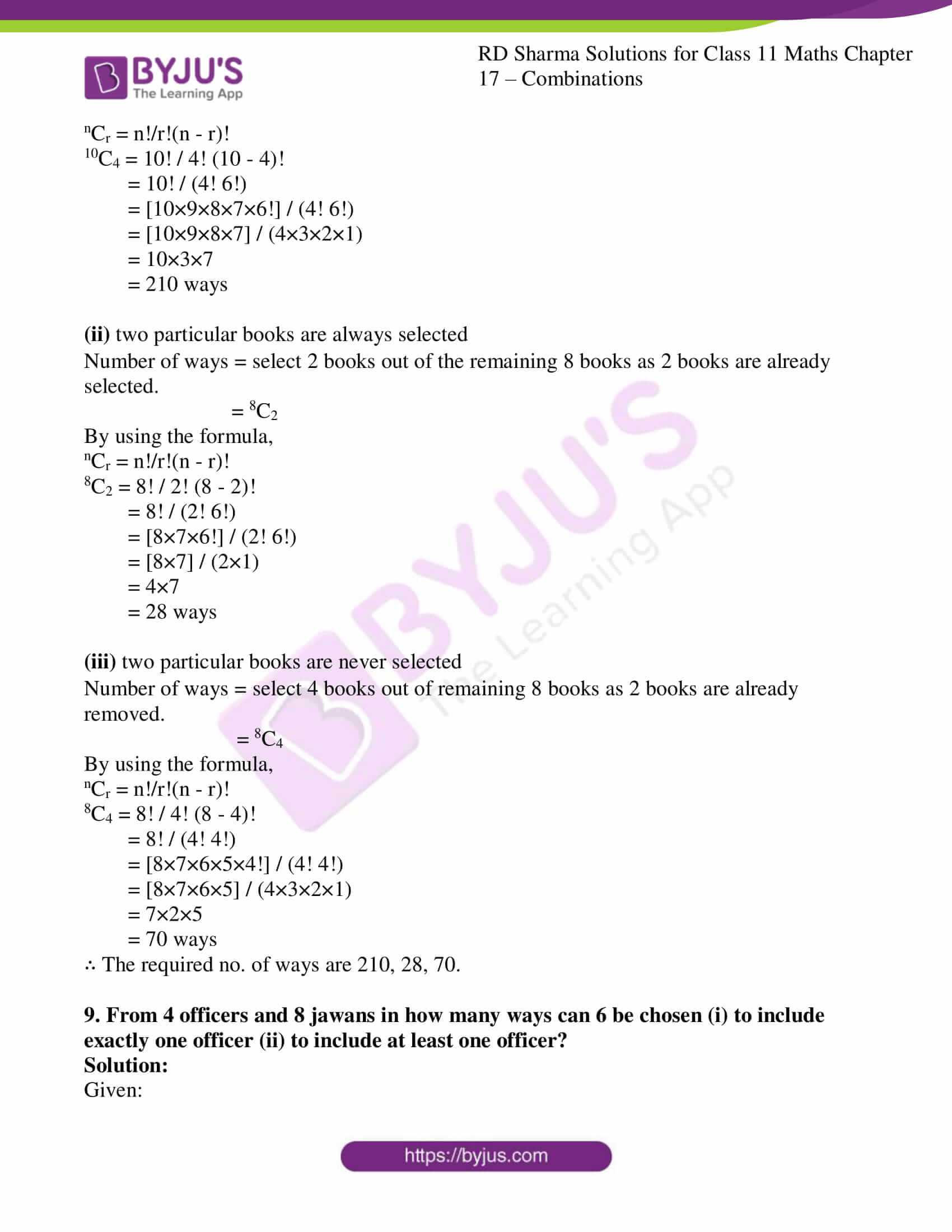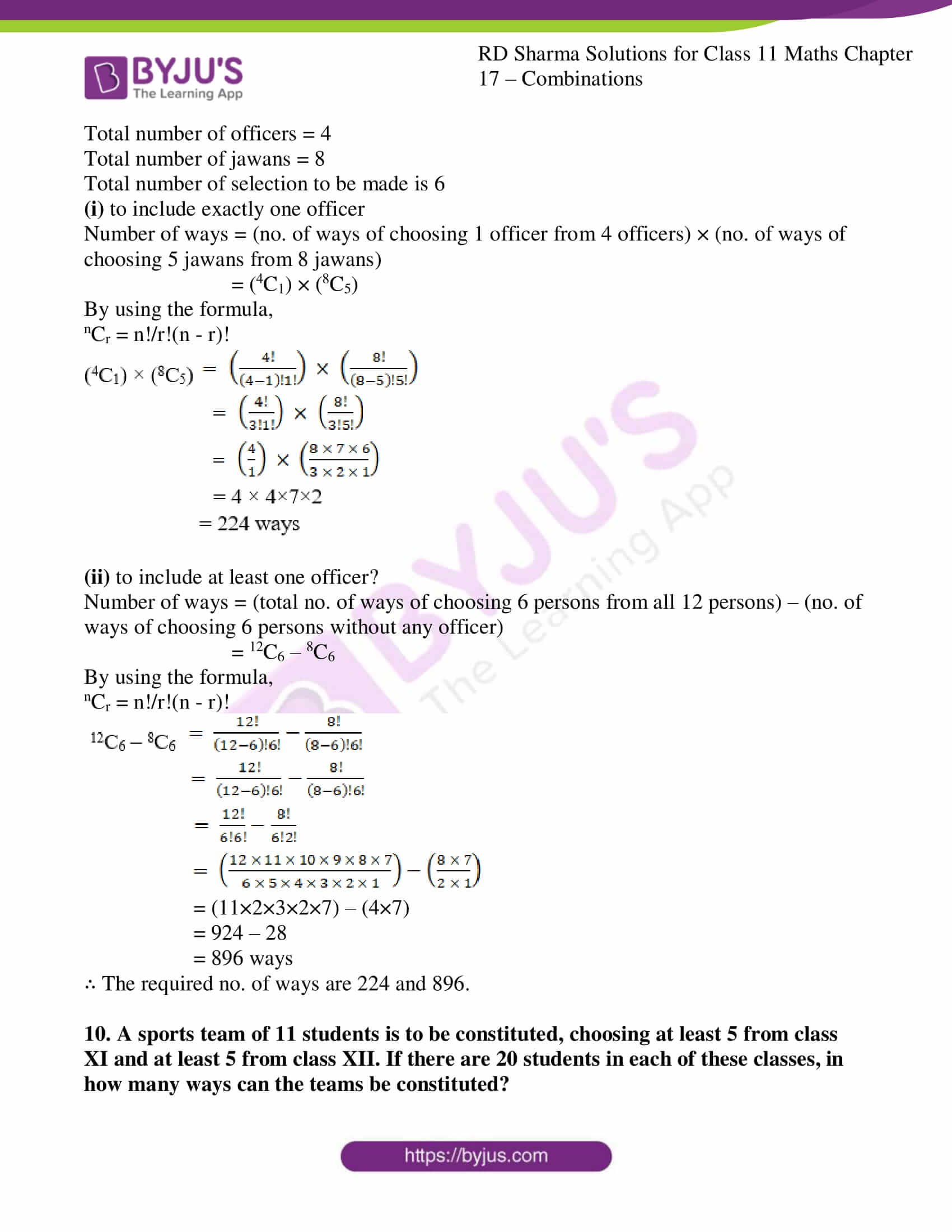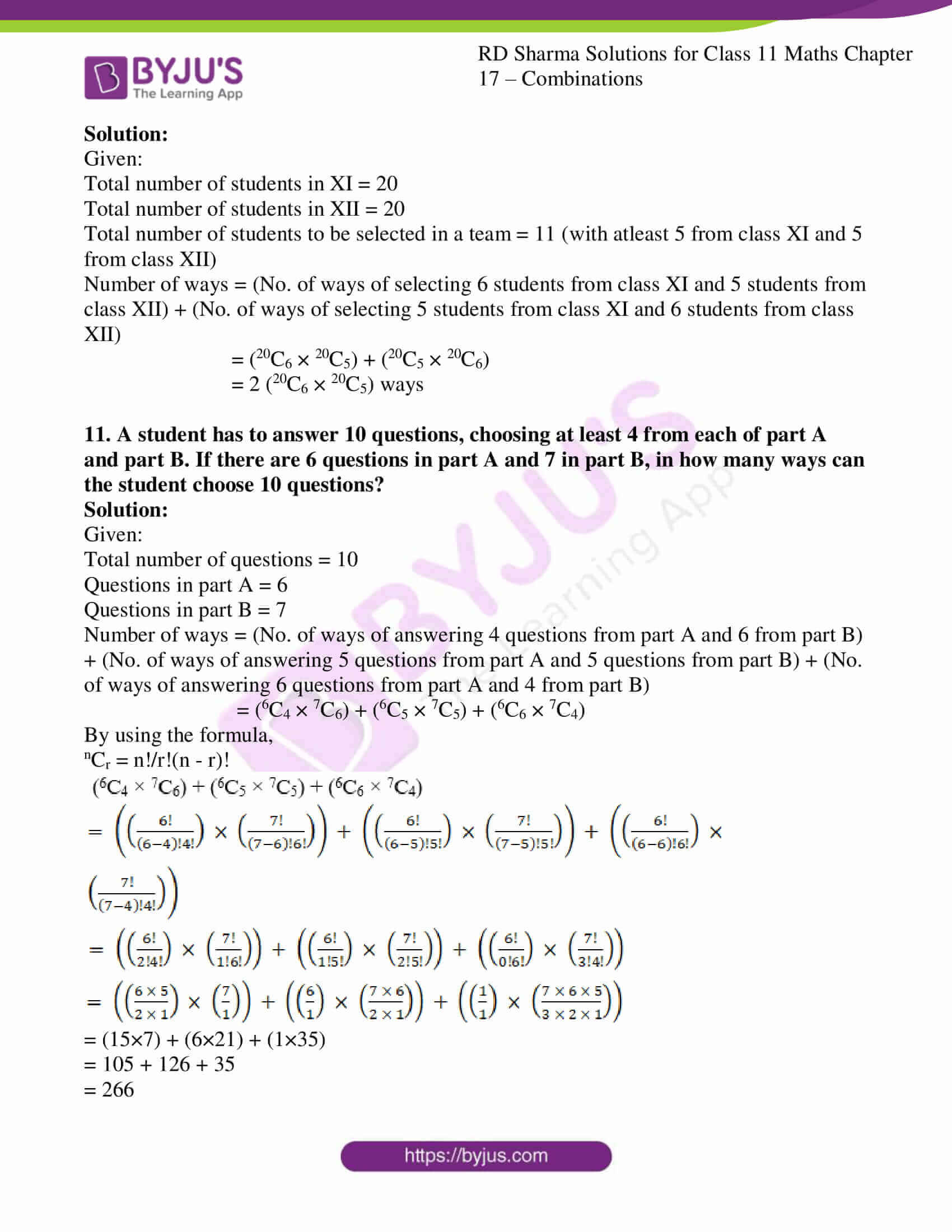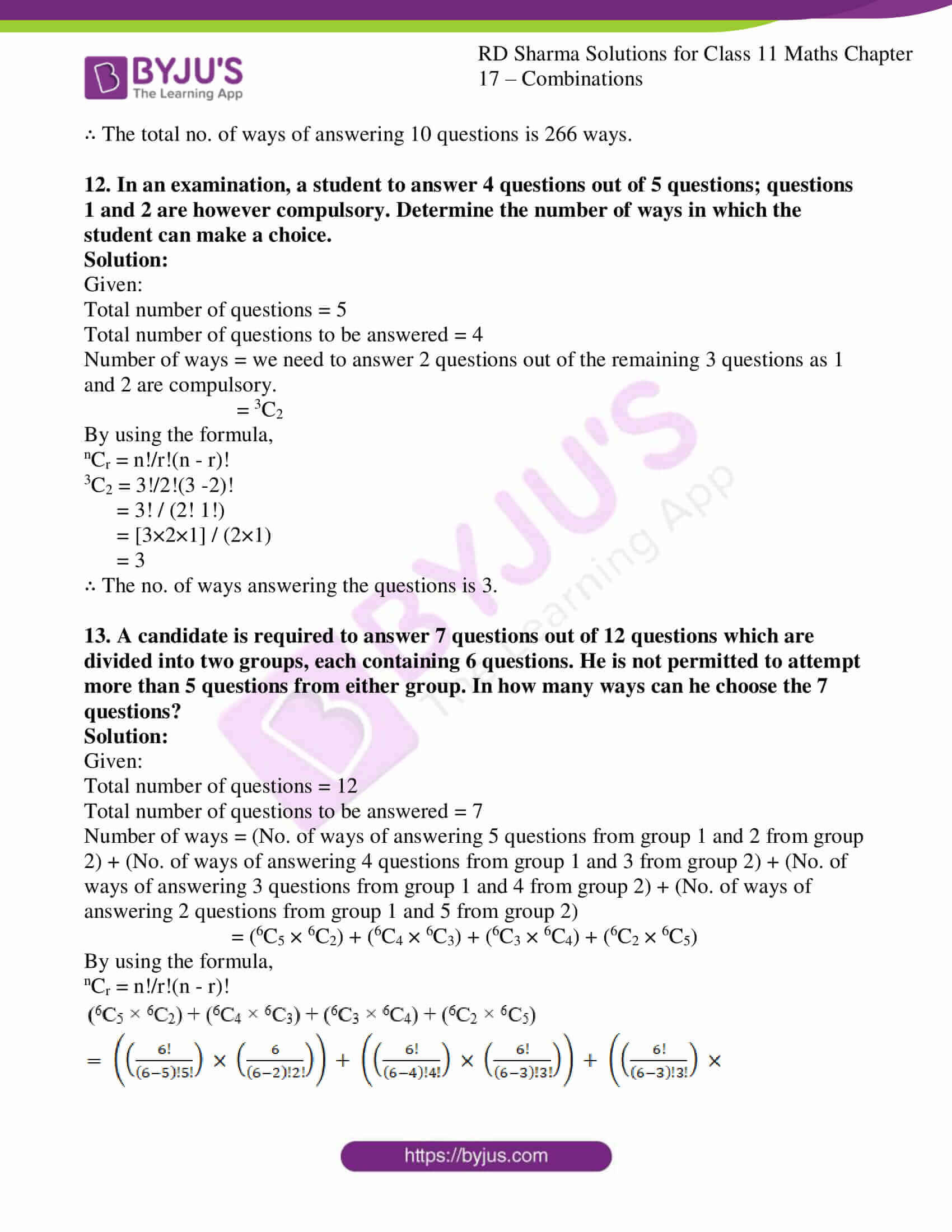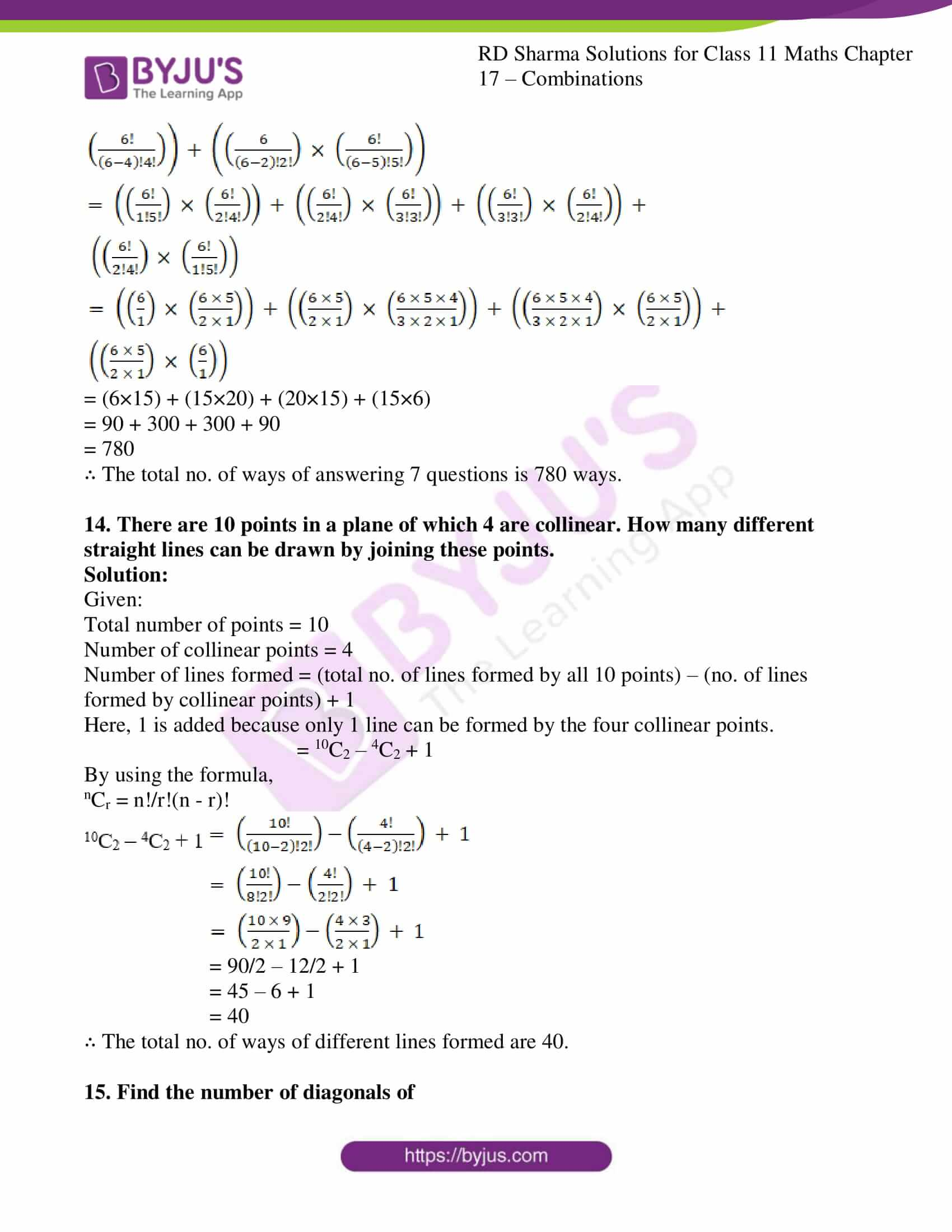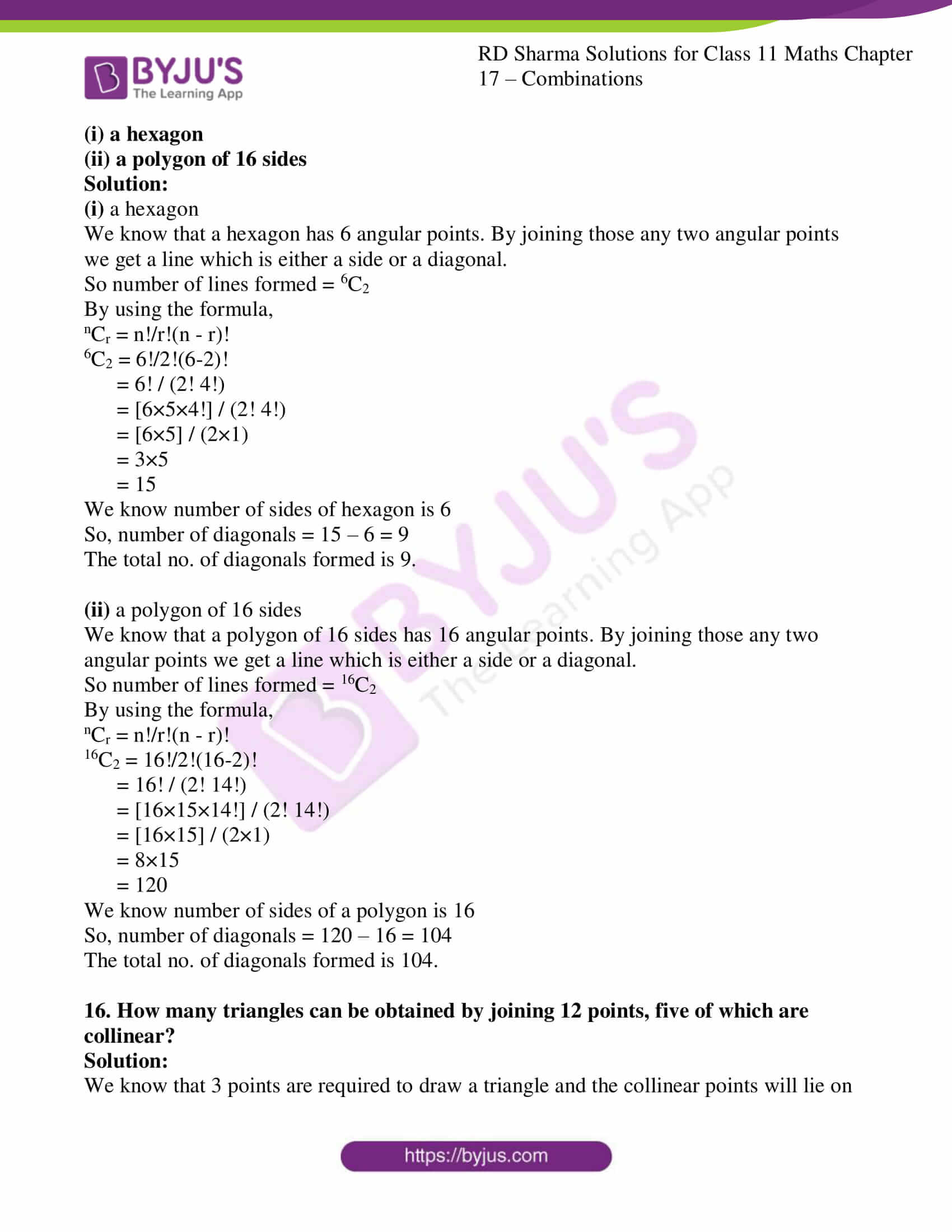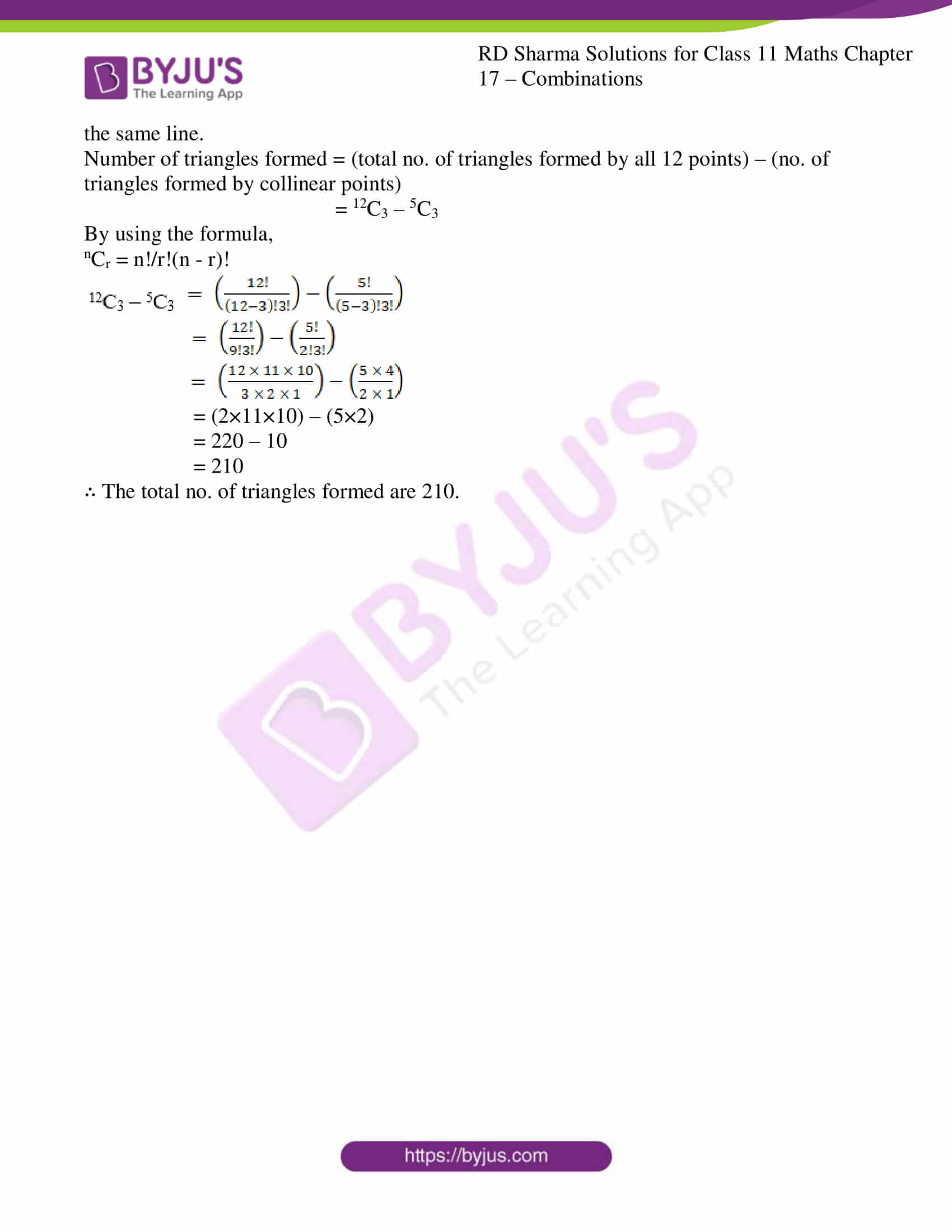### Access answers to RD Sharma Solutions for Class 11 Maths Exercise 17.2 Chapter 17 – Combinations

1. From a group of 15 cricket players, a team of 11 players is to be chosen. In how many ways can this be done?

Solution:

Given:

Number of players = 15

Number of players to be selected = 11

By using the formula,

nCr = n!/r!(n – r)!

15C11 = 15! / 11! (15 – 11)!

= 15! / (11! 4!)

= [15×14×13×12×11!] / (11! 4!)

= [15×14×13×12] / (4×3×2×1)

= 15×7×13

= 1365

∴ The total number of ways of choosing 11 players out of 15 is 1365 ways.

2. How many different boat parties of 8, consisting of 5 boys and 3 girls, can be made from 25 boys and 10 girls?

Solution:

Given:

Total boys are = 25

Total girls are = 10

Boat party of 8 to be made from 25 boys and 10 girls, by selecting 5 boys and 3 girls.

So,

By using the formula,

nCr = n!/r!(n – r)!

25C5 × 10C3 = 25!/5!(25 – 5)! × 10!/3!(10-3)!

= 25! / (5! 20!) × 10!/(3! 7!)

= [25×24×23×22×21×20!]/(5! 20!) × [10×9×8×7!]/(7! 3!)

= [25×24×23×22×21]/5! × [10×9×8]/(3!)

= [25×24×23×22×21]/(5×4×3×2×1) × [10×9×8]/(3×2×1)

= 5×2×23×11×21 × 5×3×8

= 53130 × 120

= 6375600

∴ The total number of different boat parties is 6375600 ways.

3. In how many ways can a student choose 5 courses out of 9 courses if 2 courses are compulsory for every student?

Solution:

Given:

Total number of courses is 9

So out of 9 courses 2 courses are compulsory. Student can choose from 7(i.e., 5+2) courses only.

That too out of 5 courses student has to choose, 2 courses are compulsory.

So they have to choose 3 courses out of 7 courses.

This can be done in 7C3 ways.

By using the formula,

nCr = n!/r!(n – r)!

7C3 = 7! / 3! (7 – 3)!

= 7! / (3! 4!)

= [7×6×5×4!] / (3! 4!)

= [7×6×5] / (3×2×1)

= 7×5

= 35

∴ The total number of ways of choosing 5 subjects out of 9 subjects in which 2 are compulsory is 35 ways.

4. In how many ways can a football team of 11 players be selected from 16 players? How many of these will (i) Include 2 particular players? (ii) Exclude 2 particular players?

Solution:

Given:

Total number of players = 16

Number of players to be selected = 11

So, the combination is 16C11

By using the formula,

nCr = n!/r!(n – r)!

16C11 = 16! / 11! (16 – 11)!

= 16! / (11! 5!)

= [16×15×14×13×12×11!] / (11! 5!)

= [16×15×14×13×12] / (5×4×3×2×1)

= 4×7×13×12

= 4368

(i) Include 2 particular players?

It is told that two players are always included.

Now, we have to select 9 players out of the remaining 14 players as 2 players are already selected.

Number of ways = 14C9

14C9 = 14! / 9! (14 – 9)!

= 14! / (9! 5!)

= [14×13×12×11×10×9!] / (9! 5!)

= [14×13×12×11×10] / (5×4×3×2×1)

= 7×13×11×2

= 2002

(ii) Exclude 2 particular players?

It is told that two players are always excluded.

Now, we have to select 11 players out of the remaining 14 players as 2 players are already removed.

Number of ways = 14C9

14C11 = 14! / 11! (14 – 11)!

= 14! / (11! 3!)

= [14×13×12×11!] / (11! 3!)

= [14×13×12] / (3×2×1)

= 14×13×2

= 364

∴ The required no. of ways are 4368, 2002, 364.

5. There are 10 professors and 20 students out of whom a committee of 2 professors and 3 students is to be formed. Find the number of ways in which this can be done. Further, find in how many of these committees:
(i) a particular professor is included.

(ii) a particular student is included.

(iii) a particular student is excluded.

Solution:

Given:

Total number of professor = 10

Total number of students = 20

Number of ways = (choosing 2 professors out of 10 professors) × (choosing 3 students out of 20 students)

= (10C2) × (20C3)

By using the formula,

nCr = n!/r!(n – r)!

10C2 × 20C3 = 10!/2!(10 – 2)! × 20!/3!(20-3)!

= 10!/(2! 8!) × 20!/(3! 17!)

= [10×9×8!]/(2! 8!) × [20×19×18×17!]/(17! 3!)

= [10×9]/2! × [20×19×18]/(3!)

= [10×9]/(2×1) × [20×19×18]/(3×2×1)

= 5×9 × 10×19×6

= 45 × 1140

= 51300 ways

(i) a particular professor is included.

Number of ways = (choosing 1 professor out of 9 professors) × (choosing 3 students out of 20 students)

9C1 × 20C3

By using the formula,

nCr = n!/r!(n – r)!

9C1 × 20C3 = 9!/1!(9 – 1)! × 20!/3!(20-3)!

= 9!/(1! 8!) × 20!/(3! 17!)

= [9×8!]/(8!) × [20×19×18×17!]/(17! 3!)

= 9 × [20×19×18]/(3!)

= 9× [20×19×18]/(3×2×1)

= 9 × 10×19×6

= 10260 ways

(ii) a particular student is included.

Number of ways = (choosing 2 professors out of 10 professors) × (choosing 2 students out of 19 students)

10C2 × 19C2

By using the formula,

nCr = n!/r!(n – r)!

10C2 × 19C2 = 10!/2!(10 – 2)! × 19!/2!(19-2)!

= 10!/(2! 8!) × 19!/(2! 17!)

= [10×9×8!]/(2! 8!) × [19×18×17!]/(17! 2!)

= [10×9]/2! × [19×18]/(2!)

= [10×9]/(2×1) × [19×18]/(2×1)

= 5×9 × 19×9

= 45 × 171

= 7695 ways

(iii) a particular student is excluded.

Number of ways = (choosing 2 professors out of 10 professors) × (choosing 3 students out of 19 students)

10C2 × 19C3

By using the formula,

nCr = n!/r!(n – r)!

10C2 × 19C3 = 10!/2!(10 – 2)! × 19!/3!(19-3)!

= 10!/(2! 8!) × 19!/(3! 16!)

= [10×9×8!]/(2! 8!) × [19×18×17×16!]/(16! 3!)

= [10×9]/2! × [19×18×17]/(3!)

= [10×9]/(2×1) × [19×18×17]/(3×2×1)

= 5×9 × 19×3×17

= 45 × 969

= 43605 ways

∴ The required no. of ways are 51300, 10260, 7695, 43605.

6. How many different products can be obtained by multiplying two or more of the numbers 3, 5, 7, 11 (without repetition)?

Solution:

Given that we need to find the no. of ways of obtaining a product by multiplying two or more from the numbers 3, 5, 7, 11.

Number of ways = (no. of ways of multiplying two numbers) + (no. of ways of multiplying three numbers) + (no. of multiplying four numbers)

4C2 + 4C3 + 4C4

By using the formula,

nCr = n!/r!(n – r)!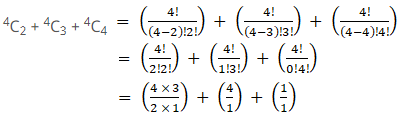= 12/2 + 4 + 1

= 6 + 4 + 1

= 11

∴ The total number of ways of product is 11 ways.

7. From a class of 12 boys and 10 girls, 10 students are to be chosen for the competition, at least including 4 boys and 4 girls. The 2 girls who won the prizes last year should be included. In how many ways can the selection be made?

Solution:

Given:

Total number of boys = 12

Total number of girls = 10

Total number of girls for the competition = 10 + 2 = 12

Number of ways = (no. of ways of selecting 6 boys and 2 girls from remaining 12 boys and 8 girls) + (no. of ways of selecting 5 boys and 3 girls from remaining 12 boys and 8 girls) + (no. of ways of selecting 4 boys and 4 girls from remaining 12 boys and 8 girls)

Since, two girls are already selected,

= (12C6 × 8C2) + (12C5 × 8C3) + (12C4 × 8C4)

By using the formula,

nCr = n!/r!(n – r)!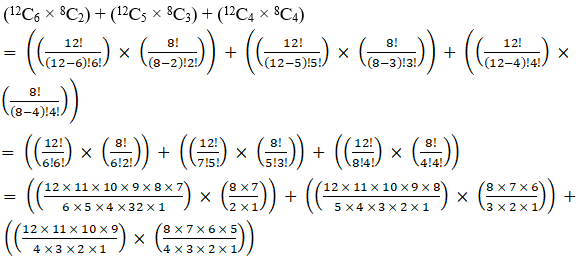= (924 × 28) + (792 × 56) + (495 × 70)

= 25872 + 44352 + 34650

= 104874

∴ The total number of ways of product is 104874 ways.

8. How many different selections of 4 books can be made from 10 different books, if
(i) there is no restriction

(ii) two particular books are always selected

(iii) two particular books are never selected

Solution:

Given:

Total number of books = 10

Total books to be selected = 4

(i) there is no restriction

Number of ways = choosing 4 books out of 10 books

10C4

By using the formula,

nCr = n!/r!(n – r)!

10C4 = 10! / 4! (10 – 4)!

= 10! / (4! 6!)

= [10×9×8×7×6!] / (4! 6!)

= [10×9×8×7] / (4×3×2×1)

= 10×3×7

= 210 ways

(ii) two particular books are always selected

Number of ways = select 2 books out of the remaining 8 books as 2 books are already selected.

= 8C2

By using the formula,

nCr = n!/r!(n – r)!

8C2 = 8! / 2! (8 – 2)!

= 8! / (2! 6!)

= [8×7×6!] / (2! 6!)

= [8×7] / (2×1)

= 4×7

= 28 ways

(iii) two particular books are never selected

Number of ways = select 4 books out of remaining 8 books as 2 books are already removed.

= 8C4

By using the formula,

nCr = n!/r!(n – r)!

8C4 = 8! / 4! (8 – 4)!

= 8! / (4! 4!)

= [8×7×6×5×4!] / (4! 4!)

= [8×7×6×5] / (4×3×2×1)

= 7×2×5

= 70 ways

∴ The required no. of ways are 210, 28, 70.

9. From 4 officers and 8 jawans in how many ways can 6 be chosen (i) to include exactly one officer (ii) to include at least one officer?

Solution:

Given:

Total number of officers = 4

Total number of jawans = 8

Total number of selection to be made is 6

(i) to include exactly one officer

Number of ways = (no. of ways of choosing 1 officer from 4 officers) × (no. of ways of choosing 5 jawans from 8 jawans)

= (4C1) × (8C5)

By using the formula,

nCr = n!/r!(n – r)!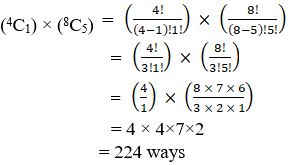(ii) to include at least one officer?

Number of ways = (total no. of ways of choosing 6 persons from all 12 persons) – (no. of ways of choosing 6 persons without any officer)

12C6 – 8C6

By using the formula,

nCr = n!/r!(n – r)!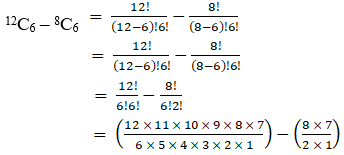= (11×2×3×2×7) – (4×7)

= 924 – 28

= 896 ways

∴ The required no. of ways are 224 and 896.

10. A sports team of 11 students is to be constituted, choosing at least 5 from class XI and at least 5 from class XII. If there are 20 students in each of these classes, in how many ways can the teams be constituted?

Solution:

Given:

Total number of students in XI = 20

Total number of students in XII = 20

Total number of students to be selected in a team = 11 (with atleast 5 from class XI and 5 from class XII)

Number of ways = (No. of ways of selecting 6 students from class XI and 5 students from class XII) + (No. of ways of selecting 5 students from class XI and 6 students from class XII)

= (20C6 × 20C5) + (20C5 × 20C6)

= 2 (20C6 × 20C5) ways

11. A student has to answer 10 questions, choosing at least 4 from each of part A and part B. If there are 6 questions in part A and 7 in part B, in how many ways can the student choose 10 questions?

Solution:

Given:

Total number of questions = 10

Questions in part A = 6

Questions in part B = 7

Number of ways = (No. of ways of answering 4 questions from part A and 6 from part B) + (No. of ways of answering 5 questions from part A and 5 questions from part B) + (No. of ways of answering 6 questions from part A and 4 from part B)

= (6C4 × 7C6) + (6C5 × 7C5) + (6C6 × 7C4)

By using the formula,

nCr = n!/r!(n – r)!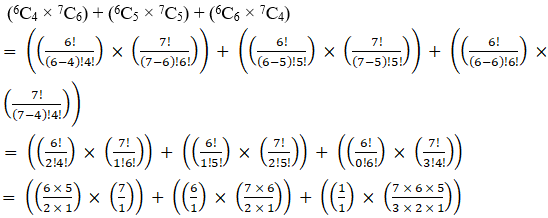= (15×7) + (6×21) + (1×35)

= 105 + 126 + 35

= 266

∴ The total no. of ways of answering 10 questions is 266 ways.

12. In an examination, a student to answer 4 questions out of 5 questions; questions 1 and 2 are however compulsory. Determine the number of ways in which the student can make a choice.

Solution:

Given:

Total number of questions = 5

Total number of questions to be answered = 4

Number of ways = we need to answer 2 questions out of the remaining 3 questions as 1 and 2 are compulsory.

= 3C2

By using the formula,

nCr = n!/r!(n – r)!

3C2 = 3!/2!(3 -2)!

= 3! / (2! 1!)

= [3×2×1] / (2×1)

= 3

∴ The no. of ways answering the questions is 3.

13. A candidate is required to answer 7 questions out of 12 questions which are divided into two groups, each containing 6 questions. He is not permitted to attempt more than 5 questions from either group. In how many ways can he choose the 7 questions?

Solution:

Given:

Total number of questions = 12

Total number of questions to be answered = 7

Number of ways = (No. of ways of answering 5 questions from group 1 and 2 from group 2) + (No. of ways of answering 4 questions from group 1 and 3 from group 2) + (No. of ways of answering 3 questions from group 1 and 4 from group 2) + (No. of ways of answering 2 questions from group 1 and 5 from group 2)

= (6C5 × 6C2) + (6C4 × 6C3) + (6C3 × 6C4) + (6C2 × 6C5)

By using the formula,

nCr = n!/r!(n – r)!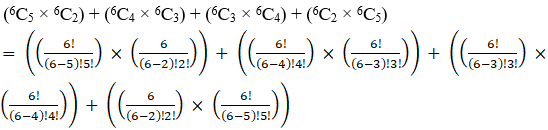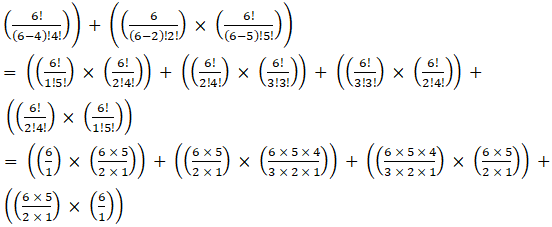= (6×15) + (15×20) + (20×15) + (15×6)

= 90 + 300 + 300 + 90

= 780

∴ The total no. of ways of answering 7 questions is 780 ways.

14. There are 10 points in a plane of which 4 are collinear. How many different straight lines can be drawn by joining these points.

Solution:

Given:

Total number of points = 10

Number of collinear points = 4

Number of lines formed = (total no. of lines formed by all 10 points) – (no. of lines formed by collinear points) + 1

Here, 1 is added because only 1 line can be formed by the four collinear points.

10C2 – 4C2 + 1

By using the formula,

nCr = n!/r!(n – r)!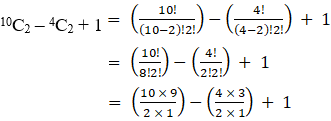= 90/2 – 12/2 + 1

= 45 – 6 + 1

= 40

∴ The total no. of ways of different lines formed are 40.

15. Find the number of diagonals of

(i) a hexagon

(ii) a polygon of 16 sides

Solution:

(i) a hexagon

We know that a hexagon has 6 angular points. By joining those any two angular points we get a line which is either a side or a diagonal.

So number of lines formed = 6C2

By using the formula,

nCr = n!/r!(n – r)!

6C2 = 6!/2!(6-2)!

= 6! / (2! 4!)

= [6×5×4!] / (2! 4!)

= [6×5] / (2×1)

= 3×5

= 15

We know number of sides of hexagon is 6

So, number of diagonals = 15 – 6 = 9

The total no. of diagonals formed is 9.

(ii) a polygon of 16 sides

We know that a polygon of 16 sides has 16 angular points. By joining those any two angular points we get a line which is either a side or a diagonal.

So number of lines formed = 16C2

By using the formula,

nCr = n!/r!(n – r)!

16C2 = 16!/2!(16-2)!

= 16! / (2! 14!)

= [16×15×14!] / (2! 14!)

= [16×15] / (2×1)

= 8×15

= 120

We know number of sides of a polygon is 16

So, number of diagonals = 120 – 16 = 104

The total no. of diagonals formed is 104.

16. How many triangles can be obtained by joining 12 points, five of which are collinear?

Solution:

We know that 3 points are required to draw a triangle and the collinear points will lie on the same line.

Number of triangles formed = (total no. of triangles formed by all 12 points) – (no. of triangles formed by collinear points)

12C3 – 5C3

By using the formula,

nCr = n!/r!(n – r)!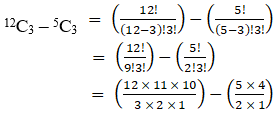= (2×11×10) – (5×2)

= 220 – 10

= 210

∴ The total no. of triangles formed are 210.